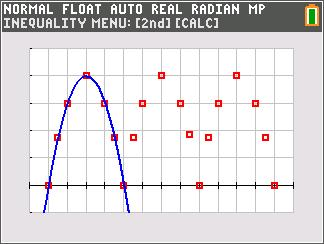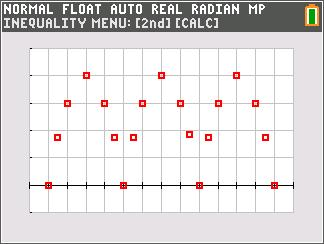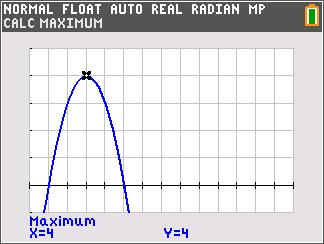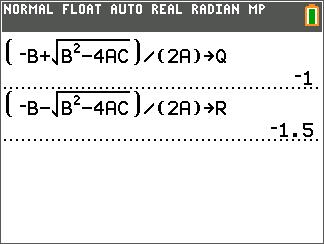••• ##### Device
• TI-84 Plus
• TI-84 Plus Silver Edition
•TI-84 Plus C Silver Edition
•TI-84 Plus CE
• ##### Software

TI Connect™ CE

• ##### Report an Issue

Algebra I: Bridge On The River Quad
by Texas Instruments#### Overview

Students graph a quadratic function that models the shape of a bridge trestle and then solve the related quadratic equation by completing the square.

#### Key Steps

•In Problem 1, students graph a given equation that models the shape of a bridge trestle.

•Students use the calculators’ capabilities to find the x-intercepts and the maximum value on the graph.

•Problem 2, allows students to use store the Quadratic Formula and use it to solve for x.

Students can also use the Equation Solver on their graphing calculator to solve for x. At the end of this activity, students will know the difference between equations and functions. They will also become familiar with several methods of finding zeros or x-intercepts.